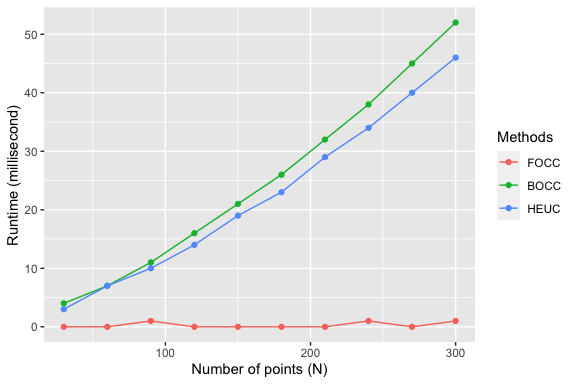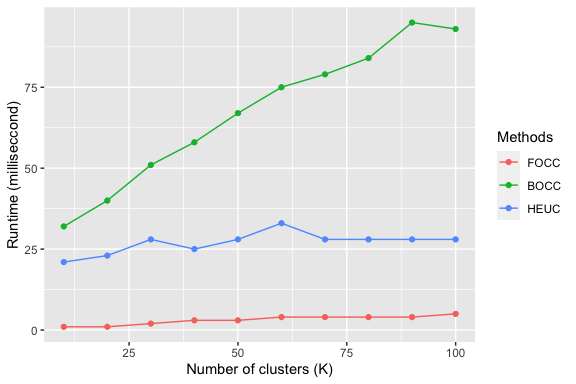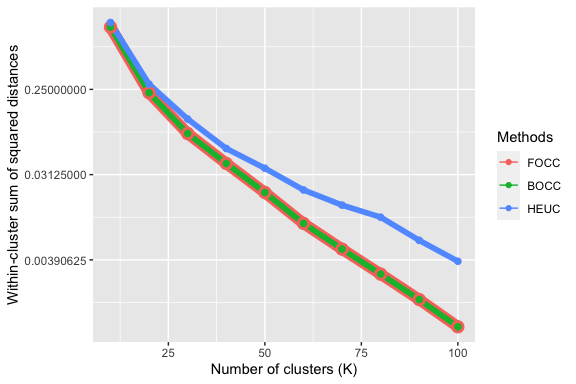# Performance of three circular data clustering algorithms

#### Updated: 2021-07-27; 2020-09-05. Created: 2020-08-07

We illustrate the runtime and quality of three circular data clustering algorithms:

1. fast optimal circular clustering (FOCC) (Debnath and Song 2021),
2. repeated heuristic $$K$$-means clustering (HEUC), and
3. brute force optimal clustering (BOCC).

## Runtime as a function of number of points for circular clustering

The runtime of both optimal and heuristic algorithms increases with the number of points in the circular data. Empirical runtime of the three algorithms as a function of the number of points $$N$$ are shown in the figure below. Both the HEUC and the BOCC algorithms increase at a quadratic rate. In contrast, the runtime of FOCC increases at a linear poly-logarithmic rate. Runtime is crucial for processing large datasets.## Runtime as a function of number of clusters

The runtime is also affected by the number of clusters $$K$$ inside the circular data. The figure below shows this effect on the three algorithms. Empirical runtime of both HEUC and BOCC increases at a similar rate to FOCC over an increasing $$K$$.## Circular clustering optimality as a function of number of clusters

The within-cluster sum of squared distances (SSQ) indicates the compactness of a cluster. A low value of SSQ suggests a good compact clustering outcome. The last figure shows the change in SSQ value with number of clusters $$K$$ in the data. The SSQ values of BOCC and FOCC always remain no more than that of the HEUC algorithm. This indicates the optimal BOCC and FOCC algorithms identify better clusters than the heuristic HEUC algorithm. The advantage of FOCC and BOCC over HEUC in SSQ gradually increases with $$K$$.## Conclusions

The examples demonstrate the advantage of FOCC algorithm over the existing BOCC and HEUC algorithms in both runtime and cluster quality. Therefore, we recommend FOCC as the best choice for circular clustering; its performance on input circular data consisting of a large number of points, having large number of clusters far exceeds the two alternatives.